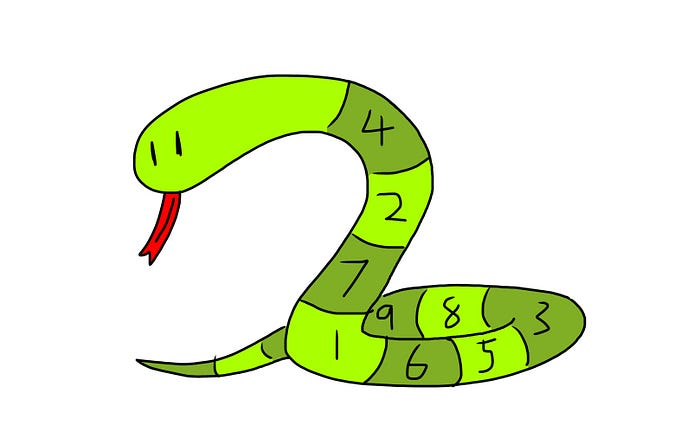# How to Sort Lists by Certain Conditions in Python

## A guide on sorting lists by a certain condition in Python.We can sort lists in Python using either the `.sort()` method.

``````list1 = [2,4,3,1]
list1.sort()
# list1 will be [1,2,3,4]
``````

However, sometimes we need to sort a list by a certain condition. For instance, let’s say we’re given a list of strings, and we need to sort it by the lengths of the strings:

``````ç
# what we want:
# lis = ["pear", "apple", "orange"]
``````

Here, `"pear"` comes first as it is the shortest string, and `"orange"` comes last as it is the longest string.

### What Sorting Normally Does

``````lis = ["apple", "orange", "pear"]
lis.sort()# lis = ["apple", "orange", "pear"]
``````

If we use the `.sort()` method normally, our list will get sorted by the default way. In this case, as we are sorting strings, our list will get sorted by alphabetical order and will remain as `["apple", "orange", "pear"]`

### How To Sort By Length Of String

``````lis = ["apple", "orange", "pear"]
lis.sort(key=len)
# lis = ["pear", "apple", "orange"]
``````

Here, notice that we added `key=len` into the arguments of `.sort`. This `key` argument is what allows us to sort the list by length.

``````lis = ["apple", "orange", "pear"]
# len("apple") -> 5
# len("orange") -> 6
# len("pear") -> 4
``````

Here, we can observe that each fruit string passed into the `len` function returns a number. This number is the length of each string and is used to sort the list.

• `"pear"` with a length of 4 is thus the smallest value
• `"apple"` with a length of 5 is the second smallest value
• `"orange"` with a length of 6 is the largest value

### The Key Argument In .sort

In `lis.sort(key=len)`, the `key` argument must be a function that takes in 1 argument. Whatever this function returns will be the condition that Python will sort our list by.

If the `key` function returns a smaller value (compared to the other elements in the list), the current element will be considered to be smaller. Here are a couple more examples

### Writing Our Own Condition For Key

``````lis = ["zaaaaa", "pineapple", "aaa"]
``````

Let’s say we’re given this list above, and we want to sort it by the number of `"a"` characters.

``````# what we want:
# lis = ["pineapple", "aaa", "zaaaaa"]
``````

Here, we need to write a function to specify this condition.

``````def condition(element):
return element.count("a")
``````

Our function here must take in 1 argument. After writing our function, we can then use our `condition` to sort our list.

``````lis.sort(key=condition)
``````

Let’s run through what’s happening here:

``````# condition("zaaaaa")     -> 5 -> largest
# condition("pineapple")  -> 1 -> smallest
# condition("aaa")        -> 3 -> second smallest
``````

And thus we get a list sorted by `condition`:

``````["pineapple", "aaa", "zaaaaa"]
``````

### A Couple More Examples

Sorting by the digit at the one’s place:

``````lis = [4,5,11,12,13]
def condition(element):
return element % 10
lis.sort(key=condition)
# lis = [11,12,13,4,5]
# condition(4)   -> 4 -> 4th element
# condition(5)   -> 5 -> 5th element
# condition(11)  -> 1 -> smallest, hence 1st element
# condition(12)  -> 2 -> 2nd element
# condition(13)  -> 3 -> 3rd element
``````

Sorting by the number of vowels:

``````lis = ["apple", "orange", "pear", "pineapple"]def condition(element):
total = 0
for vowel in "aeiou":
total += element.count(vowel)

lis.sort(key=condition)
# lis = ["apple", "pear", "orange", "pineapple"]

# condition("apple")     -> 2 vowels -> 1st
# condition("orange")    -> 3 vowels -> 3rd
# condition("pear")      -> 2 vowels -> 2nd
# condition("pineapple") -> 4 vowels -> 4th
``````

Sorting by age ( `"age"` dictionary key ):

``````lis = [
{"name":"rocky", "age":5},
{"name":"fifi", "age":3},
{"name":"remi", "age":6}
]

def condition(element):
return element["age"]

lis.sort(key=condition)

# lis = lis = [
{"name":"fifi", "age":3},
{"name":"rocky", "age":5},
{"name":"remi", "age":6}
]
``````

### Doing This In One Line Using Lambda Functions

Consider this code where we sort by the number of `"a"` characters:

``````lis = ["pineapple", "aaa", "zaaaaa"]
def condition(element):
return element.count("a")

lis.sort(key=condition)
``````

We can actually do this in one line using a lambda function:

``````lis = ["pineapple", "aaa", "zaaaaa"]
lis.sort(key=lambda x:x.count("a"))
``````

### How Lambda Functions Work

``````lambda input: output
``````

A lambda function is essentially a normal function, but we don’t need to give it a name use the `def` keyword.

``````def condition(element):
return element.count("a")
``````

does the exact same thing as:

``````condition = lambda x:x.count("a")
``````

Note that the variable `x` here is decided by us — we can use any variable name as long as it’s valid eg. `a`, `b`, `element`, etc.

### Sorting With Lambda Functions — Some Examples

Sorting by number of `"a"` characters:

``````lis.sort(key=lambda x:x.count("a"))
``````

Sorting by the digit in one’s place:

``````lis.sort(key=lambda x:x%10)
``````

Sorting by the number of vowels:

``````lis.sort(key=lambda x:sum([x.count(v) for v in "aeiou"]))
``````

Sorting by `"age"` dictionary key:

``````lis.sort(key=lambda x:x["age"])
``````

### Examples — Lambda Functions & Their Equivalent Normal Functions

Sorting by number of `"a"` characters:

``````lis.sort(key=lambda x:x.count("a"))
# SAME AS
def condition(x):
return x.count("a")
lis.sort(key=condition)
``````

Sorting by a digit in one’s place:

``````lis.sort(key=lambda x:x%10)
# SAME AS
def condition(x):
return x%10
lis.sort(key=condition)
``````

Sorting by the number of vowels:

``````lis.sort(key=lambda x:sum([x.count(v) for v in "aeiou"]))
# SAME AS
def condition(x):
return sum(x.count(v) for v in "aeiou")
lis.sort(key=condition)
``````

Sorting by `"age"` dictionary key:

``````lis.sort(key=lambda x:x["age"])
# SAME AS
def condition(x):
return x["age"]
lis.sort(key=condition)
``````

### Some Final Words

If lambda functions scare you for now, just stick with normal functions and use them to sort your list, and come back to lambda functions when you’re more comfortable. They are essentially the same thing — just that we can do lambda stuff in one line. Hope this article was helpful!